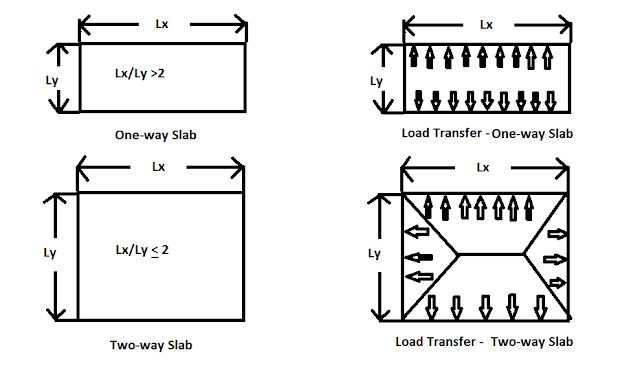# Difference Between One way and Two way Slab

### What is the Slab?

In reinforced concrete construction, the slab is an extensively used structural element forming floors and roofs. A concrete slab is the plane element having the depth d much smaller than its span and width

It may be supported by reinforced concrete beams, by masonry wall or directly by column. Difference between the one-way slab and two-way slabs are as follows :

### What is a One-way Slab?

Slab supported only on two opposite sides by rigid supports carry the load by flexure in the direction perpendicular to the supports. The plane surface of a predominantly uniformly loaded slab deforms into a cylindrical surface in which curvature and hence bending moment develops only in one direction such slabs are called a one-way slab.

### What is Two-way Slab?

The rectangular slabs supported on two adjacent, three or four edges, and with the ratio of longer to shorter span less than two, which carry the load by flexure in two perpendicular directions are called two-way slabs.

How do You calculate a one way, two-way slab?

If L/b ratio is greater than or equal 2 or then it is considered a one-way slab.

If L/b ratio is less than 2 then it is considered a two-way slab.Difference Between One way and Two-way Slab

### Difference Between One-way Slab and Two-way Slab

 S.No One-way Slab Two-way Slab 1 If L/b the ratio is greater than or equal 2 or then it is considered a one-way slab. If L/b the ratio is less than 2 then it is considered a two-way slab. 2 In one-way slab, the main reinforcement is provided in a short span and distribution reinforcement is provided in a long span. In two-way slab, the main reinforcement is provided in both directions. 3 In one-way slab, the crank is provided in two directions. In two-way slab, the crank is provided in four directions. 4 The one-way slab is supported by a beam on two opposite side only. The two-way slab is supported by the beam on all four sides. 5 In one-way slab, the load is carried in one direction perpendicular to the supporting beam. In two-way slab, the load is carried in both directions. 6 The deflected shape of the one-way slab is cylindrical. Whereas the deflected shape of the two-way slab is a dish or saucer-like shape. 7 Chajja and Varandha are practical examples of one-way slab Whereas two-way slabs are used in constructive floors of the Multistorey building. 8 While designing one-way slab we provide less steel hence the depth of the slab increases, as a result, the thickness of the one-way slab is more as compared to the two-way slab. While designing two-way slab we provide more steel hence the depth of slab decreases, as a result, the thickness of two-way slab is less as compared to the one-way slab. 9 The one-way slab is economical up to a span of 3.6 meters. Whereas the two-way slab is economical for the panel sizes up to 6m × 6m. 10 In one-way slab quantity of steel is less. In two-way slab quantity of steel is more as compared to the one-way slab. 11 In one-way slab, bending is only in one direction i.e. in a shorter span. In a two-way slab, bending is in both directions.

In practices, the choice of the one-way slab and two-way slab for a particular structure will largely depend upon the

1. Economy
2. Buildability
4. length of the span

So, friends, I hope I have covered all the differences between the one-way slab and two-way slab in this article. If you find this article helpful please be sure to share it with someone who might benefit from it. If you want to add any information which has missed in this article you can mention it in the comment section.